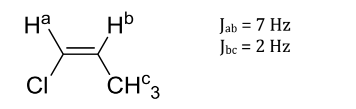# Problem: Use the following molecule and coupling constants for this problem. Draw the splitting tree that models the 1H NMR coupling, for Hb, based on the coupling constants (J) above. Label the coupling constants (in Hz) for each split of the peaks, just as they were labeled in your textbook.

###### FREE Expert Solution
80% (376 ratings)
###### Problem Details

Use the following molecule and coupling constants for this problem.

Draw the splitting tree that models the 1H NMR coupling, for Hb, based on the coupling constants (J) above. Label the coupling constants (in Hz) for each split of the peaks, just as they were labeled in your textbook.Frequently Asked Questions

What scientific concept do you need to know in order to solve this problem?

Our tutors have indicated that to solve this problem you will need to apply the 1H NMR: Spin-Splitting Complex Tree Diagrams concept. You can view video lessons to learn 1H NMR: Spin-Splitting Complex Tree Diagrams. Or if you need more 1H NMR: Spin-Splitting Complex Tree Diagrams practice, you can also practice 1H NMR: Spin-Splitting Complex Tree Diagrams practice problems.

What is the difficulty of this problem?

Our tutors rated the difficulty ofUse the following molecule and coupling constants for this p...as high difficulty.

How long does this problem take to solve?

Our expert Organic tutor, Jonathan took 6 minutes and 26 seconds to solve this problem. You can follow their steps in the video explanation above.